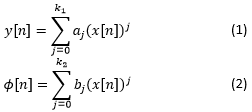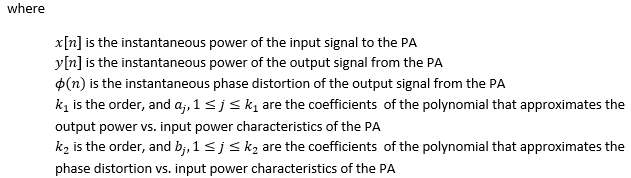# Memoryless DPD

NI-RFmx SpecAn 3.0 Help

Edition Date: January 2019

Part Number: 374264L-01

»View Product InfoDownload Help (Windows Only)

## Lookup Table Based DPD

PAs with negligible memory effects can be easily linearized by suitably modifying or predistorting amplitude and phase of the complex baseband representation of the input signal. Lookup table (LUT) based DPD assumes that the instantaneous output power from the PA and the phase distortion introduced by the PA are nonlinear functions of the instantaneous input signal power.

The input-output characteristics of a non-linear PA can be approximated by using a polynomial as shown in the following equations:LUT based digital predistortion coefficients can be computed in a logarithmic or a linear scale. The first step is obtaining the output power (dBm or watts) and phase distortion (degrees) vs. input power (dBm or watts) characteristics of the PA as expressed in equations (1) and (2) respectively. In the second step, we use these characteristics to create a predistortion lookup table which consists of complex correction factors and corresponding input powers (dBm or watts). The input powers in the lookup table are equally spaced in logarithmic or linear scale.